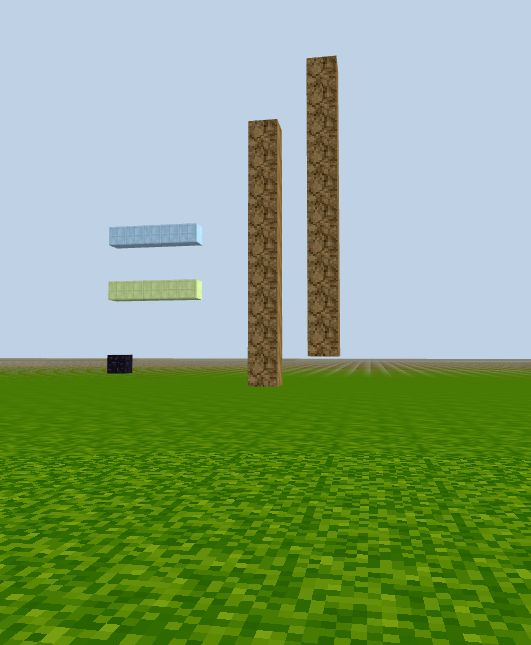### Build vertical columns

Just like the row of blocks you created in last lesson, you can also create vertical columns or rows in the z-direction by looping through y and z values.

Column:

``````for (let j = 0; j < 10; j++) {
game.setBlock(new Position(10, j, -10), 20)
}
``````

The loop counter `j` receives values `0,1,2,...,9`

`for` loop will iterate through these numbers, and for each of them, run the code under the `for loop` header line -- call the function `game.setBlock()` to lay a block at coordinates `(10, j, -10)`.

After the loop is over, there will be 10 blocks total at locations:
`(10,0,-10), (10,1,-10), (10,2,-10),..., (10,9,-10)`

Together, they make up a column of 10 blocks tall at the horizontal location `(x=10, z=-10)`. (Even though this column is 10 blocks tall, we can only see 9 blocks above the ground because the first block at `y=0` is buried in the ground).

It's easy to shift the column up to show all the blocks by changing the loop counter value to be

`let j = 1; j < 11; j++`

``````for (let j = 1; j < 11; j++) {
game.setBlock(new Position(10, j, -10), 20)
}
``````